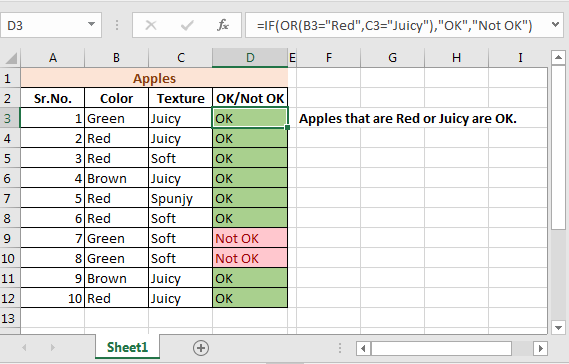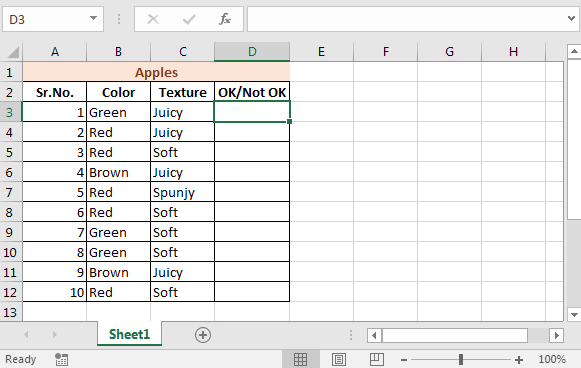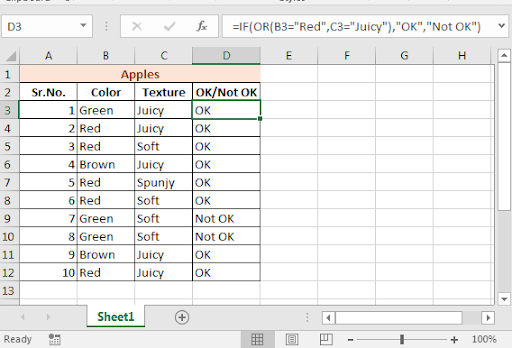# IF with OR Function in Excel

A fruit seller is selling apples. You will buy apples only if they are Red or Juicy. If an apple is not Red nor Juicy, you will not buy it.Here we have two conditions and at least one of them need to be true to make you happy. Let’s write an IF OR formula for this in Excel 2016.
Implementation of IF with OR
Generic Formula

=IF(OR(condition1, condition2,...),value if true, value if false)

Example
Let's consider the example we discussed in the beginning.
We have this table of apple’s colour and type.
If the colour is “Red” or type is “Juicy” then write OK in column D. If the color is not “Red”, nor the type is “Juicy” then type Not OK.Write this IF OR formula in D2 column and drag it down.

=IF(OR(B3="Red",C3="Juicy"),"OK","Not OK")

And you can see now that only apples that are Red or Juicy are marked OK.How It Works
IF Statement : You know how IF function in Excel works. It takes a boolean expression as first argument and returns one expression if TRUE and another if FALSE. Learn more about The Excel  IF function.

=IF(TRUE or FALSE, statement if True, statement if false)

OR Function: Checks multiple conditions. Returns TRUE only if at least one of the conditions is TRUE else returns FALSE.

=OR(condition1, condition2,....) ==> TRUE/FALSE

In the end, OR function provides IF function TRUE or FALSE argument and based on that IF prints the result.
Alternate Solution:
Another way to do this is to use nested IFs for Multiple Conditions.

=IF(B3="Red", “OK”, IF(C3="Juicy",”OK”,”Not OK”),”Not OK”)

Nested IF is good when we want different results but not when only one result. It will work but for multiple conditions, it will make your excel formula too long.

So here we learned about how to use IF with OR to check multiple conditions and show results if at least one of all conditions is TRUE. But what if you want to show results only if all condition is true. We will use AND function with IF in excel to do so.

Related Articles:

Excel OR function

Excel AND Function

IF with AND Function in Excel

Excel TRUE Function

Excel NOT function

IF not this or that in Microsoft Excel

IF with AND and OR function in Excel

Popular Articles:

The VLOOKUP Function in Excel

COUNTIF in Excel 2016

How to Use SUMIF Function in Excel

Terms and Conditions of use

The applications/code on this site are distributed as is and without warranties or liability. In no event shall the owner of the copyrights, or the authors of the applications/code be liable for any loss of profit, any problems or any damage resulting from the use or evaluation of the applications/code.